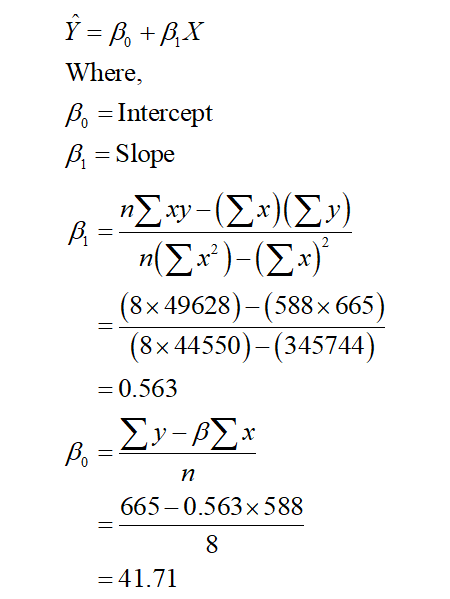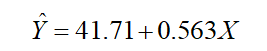# A research hospital did a study on relationship between stress and diastolic blood pressure. the results from eight patients in the study are given in the table below. The units for blood pressure values are measured in mililiters of mercury.Stress test score, x values   55 62 58 78 92 88 75 80Blood pressure, y  values    70 85 72 85 96 90 82 851 Find the regression line equation for this data. 2 Use the regression equation to estimate the diastolic blood pressure of a person whose stress test score was 85.3 What is the meaning of the slope of the regression line in the context of the problem

Question
1 views

A research hospital did a study on relationship between stress and diastolic blood pressure. the results from eight patients in the study are given in the table below. The units for blood pressure values are measured in mililiters of mercury.

Stress test score, x values   55 62 58 78 92 88 75 80
Blood pressure, y  values    70 85 72 85 96 90 82 85

1 Find the regression line equation for this data.

2 Use the regression equation to estimate the diastolic blood pressure of a person whose stress test score was 85.

3 What is the meaning of the slope of the regression line in the context of the problem

check_circle

Step 1

The regression is a linear relationship between one dependent variable (Y) and one or more than one independent variable (X).

1)

The regression line can be defined as:Therefore, the linear regression line becomes,Where Y is the blood pressure of a person and X is the stress test score.

...

### Want to see the full answer?

See Solution

#### Want to see this answer and more?

Solutions are written by subject experts who are available 24/7. Questions are typically answered within 1 hour.*

See Solution
*Response times may vary by subject and question.
Tagged in

### Statistics